Hu, Z., Zhang, G., Chen, Y., Wang, Y., He, Y., Peng, L., and Wang, L. (2018). "Determination of lignin monomer contents in rice straw using visible and near-infrared reflectance spectroscopy," BioRes. 13(2), 3284-3299.

#### Abstract

Genetic modification of plant lignin composition is an important strategy for improving biomass enzymatic digestibility without sacrificing the normal growth of the plant. However, the absence of fast and convenient methods for rapid determination of lignin composition has impeded corresponding research. Near-infrared reflectance spectroscopy (NIRS) analysis has potential as a solution for this dilemma, while the NIRS measurement for expediently assaying lignin composition in rice straws is still lacking. In this study, visible and near-infrared reflectance spectroscopy (VIS/NIRS) and modified partial least squares (MPLS) method were combined to develop calibration models for predicting the lignin monomer contents in a diverse rice population. Four optimal equations for predicting the content of p-hydroxyphenyl (H), guaiacyl (G), and syringyl (S) lignin units and their total amount (H + S + G) were generated with acceptable determination coefficients for calibration (0.85 to 0.93), cross-validation (0.75 to 0.88), and external validation (0.82 to 0.88), and the ratio performance deviation (RPD, 2 to 3.01). This study was the first to demonstrate that VIS/NIRS could give a sufficiently accurate prediction of lignin monomer contents in rice and could be applied for rapid assessments of large-scale rice straw samples.

Determination of Lignin Monomer Contents in Rice Straw Using Visible and Near-infrared Reflectance Spectroscopy

Zhen Hu,a,b,c Guifen Zhang,a,b,c Yuanyuan Chen,a,b,c Youmei Wang,a,b,c Yuqing He,b,d Liangcai Peng,a,b,c and Lingqiang Wang a,b,c,*

Genetic modification of plant lignin composition is an important strategy for improving biomass enzymatic digestibility without sacrificing the normal growth of the plant. However, the absence of fast and convenient methods for rapid determination of lignin composition has impeded corresponding research. Near-infrared reflectance spectroscopy (NIRS) analysis has potential as a solution for this dilemma, while the NIRS measurement for expediently assaying lignin composition in rice straws is still lacking. In this study, visible and near-infrared reflectance spectroscopy (VIS/NIRS) and modified partial least squares (MPLS) method were combined to develop calibration models for predicting the lignin monomer contents in a diverse rice population. Four optimal equations for predicting the content of p-hydroxyphenyl (H), guaiacyl (G), and syringyl (S) lignin units and their total amount (H + S + G) were generated with acceptable determination coefficients for calibration (0.85 to 0.93), cross-validation (0.75 to 0.88), and external validation (0.82 to 0.88), and the ratio performance deviation (RPD, 2 to 3.01). This study was the first to demonstrate that VIS/NIRS could give a sufficiently accurate prediction of lignin monomer contents in rice and could be applied for rapid assessments of large-scale rice straw samples.

Keywords: Visible and near-infrared reflectance spectroscopy (VIS/NIRS); Calibration models; Lignin monomer; Rice

Contact information: a: Biomass and Bioenergy Research Centre, Huazhong Agricultural University, Wuhan, China; b: National Key Laboratory of Crop Genetic Improvement, Huazhong Agricultural University, Wuhan, China; c: College of Plant Science and Technology, Huazhong Agricultural University, Wuhan, China; d: College of Life Science and Technology, Huazhong Agricultural University, Wuhan, China; *Corresponding author: lqwang@mail.hzau.edu.cn

INTRODUCTION

Lignin is a crucial material in the evolution of plants from an aquatic environment to a land environment and provides land plants water transport, mechanical support, stress response, and pathogen resistance (Boerjan et al. 2003). Unfortunately, it has been identified as a major contributor to biomass recalcitrance, which is the blockage that leads to the high cost of lignocellulosic sugar production (Ding et al. 2012; Zeng et al. 2014). This economic effect has promoted lignin modification to become a popular research topic. Numerous studies have established that increased biomass digestibility could be gained by reduced lignin content and altered lignin composition (Fu et al. 2011; Bonawitz et al. 2014). However, a reduction in lignin often disturbs plant development and causes undesirable effects (Chen and Dixon 2007; Bonawitz and Chapple 2013).

A feasible method for lignin engineering to decrease biomass recalcitrance involves changing the proportion of three major types of lignin units, p-hydroxyphenyl (H), guaiacyl (G), and syringyl (S) units (Wang et al. 2016).

The lignin monomer content is typically a quantitative trait, while the studies that attempted to identify monolignol synthesis genes through quantitative trait locus/loci (QTL) mapping or genome-wide association study (GWAS) have been very limited (BarrièRe et al. 2008). A main reason that limits the use of classic quantitatively genetic methods in identification of the monolignol synthesis gene might be the complex process for assaying lignin monomer content on a large scale.

Traditional wet chemical methods used to analyze lignin composition include thioacidolysis (TA), nitrobenzene oxidation (NBO), and derivatization followed by reductive cleavage (DFRC) (Wen et al. 2013). Unfortunately, all of these methods are labor-intensive and time-consuming. Even though high-throughput systems with small vials have been developed to improve the efficiency of the process (Foster et al. 2010; Penning et al. 2014), additional costs of the equipment might limit their applications to some extent. Nonetheless, reliable, low-cost, and time-effective methods for lignin composition assessments are urgently needed.

The NIRS analysis has been shown to be a fast, environmentally friendly analytical method and has gained a wide variety of applications in the prediction of biomass property and component composition (Xu et al. 2013). The absorption of the near-infrared spectroscopy radiation is primarily induced by overtone and combination bands of fundamental stretching vibrations of O-H, C-H, and N-H, which represent the major chemical bonds in biological compounds (Bailleres et al. 2002). Through mathematical approaches, near-infrared spectral data, and reference parameters are combined to build the calibration models (Payne and Wolfrum 2015). Calibration models for accurate prediction of the biomass compositions of rice (Jin and Chen 2007), wheat (Owens et al. 2009), sorghum (Wu et al. 2015; Xu et al. 2015), Miscanthus (Jin et al. 2017), bamboo (Yang et al. 2016), sugarcane (Hoang et al. 2017), and wood (Bailleres et al. 2002), have been built using NIRS. However, the progress in quantitative prediction of lignin composition in plants is still very limited, and all of these studies were conducted on just wood (Bailleres et al. 2002; Alves et al. 2006; Yamada et al. 2006; Maranan and Laborie 2008; Robinson and Mansfield 2009).

Rice is an important food crop for human consumption. Its annual production generates about 800 million metric tons of straw, which provides an abundant biomass source for the production of renewable energy (Domínguez-Escribá and Porcar 2010). A reliable and fast method for assaying lignin composition of rice straw is necessary to efficiently design and screen rice varieties that are more amenable to enzymatic digestibility. Up until now, no research has been conducted to build NIRS calibration equations for the prediction of lignin composition in rice materials. The target of this work was to establish a rapid and accurate VIS/NIRS measurement for expediently assaying lignin composition in rice straw.

EXPERIMENTAL

Materials

The 100 rice straw samples were selected from a rice recombinant inbred line (RIL) population described by Yan et al. (2014). The rice lines were planted in the experimental field of Huazhong Agricultural University (Wuhan, China) during the natural growing season in 2012. The straws of three individual plants for each line were collected after grain harvest. Then the leaves were removed, and the stems were inactivated at 105 C for 30 min and dried at 60 C to a constant weight. The dried stems were ground to pass through a 40-mesh screen and stored in a dry container until their use.

Methods

Cell wall extraction and lignin composition assay

Cell wall isolation and lignin composition assay were conducted using a high-throughput platform previously described by Foster et al. (2010). The isolated cell wall material was prepared by washing the samples with 1.5 mL of 70% aqueous ethanol and 1.5 mL of chloroform/methanol (1:1, v/v), followed by treatment with 35 μL of 0.01% sodium azide (NaN3), 35 μL amylase (50 μg/mL in H2O, from Bacillus species, Sigma), and 17 μL pullulanase (17.8 units, from Bacillus acidopullulyticus, Sigma). For lignin composition assay, 2 mg of isolated cell wall material was transferred into a screw-capped glass tube. Dioxane (175 μL), ethanethiol (EtSH, 20 μL 10%), and boron trifluoride diethyl etherate (BF3, 5 μL 2.5%) were added for thioacidolysis. The reaction was heated at 100 C for 4 h with gentle mixing every hour. For purification, 1 mL of water and 0.5 mL of ethyl acetate was added, then mixed thoroughly with a vortex mixer, and the phases were left to separate. Next, 150 μL of the ethyl acetate layer (top layer) was transferred to a 2 mL Sarstedt tube.

The solvent was evaporated by a concentrator with air. For the trimethylsilyl (TMS) derivatization, 500 μL of ethyl acetate, 20 μL of pyridine, and 100 μL of N, O-bis (trimethylsilyl) acetamide were added together, and the mixture was incubated for 2 h at 25 ℃. The reaction products (100 μL) were analyzed by gas chromatography (GC) with a quadrupole mass-spectrometer (Santa Clara, CA, USA) or flame ionization detector (Restek Corporation, Bellefonte, PA, USA). Peaks were identified by characteristic mass spectrum ions of 299 m/z, 269 m/z, and 239 m/z for S, G, and H monomers, respectively. The composition of the lignin components was quantified by setting the total peak area to 100%. The chemical agents were purchased from Sigma-Aldrich, St. Louis, MO, USA.

Near-infrared reflectance spectra data collection and analysis

Spectra collection and variability analysis of NIRS were conducted as described by Huang et al.(2012). Near-infrared reflectance spectra data was collected using a XDS Rapid Content™ Analyzer (FOSS, Co., LLC., Hillerod, Denmark) equipped with a dual detector system: silicon (400 nm to 1100 nm), lead sulfide (1100 nm to 2500 nm), and the ISIscan™ software (Infrasoft International LLC., Port Mathilda, PA, USA). The dried samples were placed into a mini-sample cup (standard ring cup) for screening. The reflectance (R) of each sample was recorded in triplicate at wavelengths ranging from 400 nm to 2500 nm with 2-nm intervals at room temperature.

The spectral absorbance values were recorded as log1/R. The WinISI III software package (Version 1.50e, Infrasoft International LLC., Port Matilda, PA, USA) was used for the chemometric management of data. A principal component analysis (PCA) algorithm was carried out to identify the spectral outlier sample and determine the structure and variability of spectral population (Cowe and McNicol 1985). The global H (GH) value of each sample was determined using the measured Mahalanobis distance from mean. The GH outlier (GH > 3.0) was finally eliminated after the PCA. Full spectra wavelengths (408 nm to 2492 nm) were selected and pretreated with “1, 4, 4, 1” treatments for principal component analysis, the four digits orderly represented the number of the derivative, the gap over which the derivative is calculated, the number of the first smoothing, and the number of the second smoothing.

Calibration and validation

The prediction models were calibrated and validated following the procedure of Huang et al. (2012) with minor modifications. Two mathematical treatments as “0, 0, 1, 1” and “1, 4, 4, 1”, and seven scatter correction methods were used to build prediction equations.

The seven methods were: no scatter correction standard (None), standard normal variant (SNV), detrend only (DET), combination of SNV and detrend (SNVD), standard multiple scatter correction (SMSC), weighted multiple scatter correction (WMSC), and inverse multi scatter correction (IMSC). In addition, three wavelength ranges (408 nm to 2492 nm, 780 nm to 2492 nm, and 1108 nm to 2492 nm), and the modified partial least squares (MPLS) method were also utilized.

Cross-validation was conducted during the development of equations to select the optimal number of factors and to avoid over-fitting. In addition, one of every four samples sorted based on the laboratory value were selected for the external validation. The coefficient of determination of the cross-validation (R2cv) was defined as the reference index to select the best equations.

QTL mapping using measured data and predicted data

The genetic map of the recombinant inbred line (RIL) population was built using 179 markers and MAPMAKER/EXP version 3.0b software (Whitehead Institute for Biomedical Research, Cambridge, MA, USA). The following inclusive composite interval mapping was carried out using QTL IciMapping version 3.3 software (Institute of Crop Science, Chinese Academy of Agricultural Sciences, Beijing, China) based on stepwise regression with simultaneous consideration of all marker information. The walking speed chosen for all QTL mapping was 1.0 cM.

Statistical analysis

A statistical analysis for calculating the minimum, maximum, mean, and standard deviation and the Kolmogorov-Smirnov test for checking the normal distribution were conducted using the software of the SPSS 17.0 (SPSS Inc., Chicago, IL, USA). The best-fit curve in frequency distributions and descriptors of regression analysis were developed using Origin 8.0 software (Microcal Software, Northampton, MA).

RESULTS AND DISCUSSION

Characterization of Spectra and Identification of Outlier

The averaged VIS/NIR spectrum of 100 rice samples with three replications was generated by the WinISI III software and is shown in Fig. 1. There was one absorption band peak that occurred in the visible region (400 nm to 770 nm) and six peaks that occurred in the near infrared region (780 nm to 2500 nm).

The strong peak in the visible region at 438 nm might have been induced by the yellow-orange color of the rice powders, as the spectral region from 425 nm to 492 nm represents the complementary color of yellow-orange (Shenk and Westerhaus 1994). The peak at 1216 nm and 1492 nm is primarily attributed to the 2nd and 1st overtone O–H stretching in cellulose, respectively (Schwanninger et al.2011). The peak at 1928 nm is mainly attributed to the asymmetric stretching and deformation of O–H in water (Ali et al. 2001). The broad peak at 2110 nm is associated with the stretching and deformation of O–H in cellulose (Schwanninger et al. 2011). The peak at 2278 nm could be assigned to the O-H and C-C stretching and/or C-H stretching and deformation in cellulose (Ali et al. 2001). The last peak at 2336 nm is mainly attributed to the C-H stretching and C-H2 deformation in lignin (Schwanninger et al. 2011). The original spectra and the pretreated spectra dealt with six scatter methods and the first-order derivative of all samples were also exhibited (Fig. 2).

The peaks in the pretreated spectra were clearer and steeper than those in original spectra and this might have been because the math pretreatments decreased the interferences induced by particle size, scatter coefficient, and path length variation.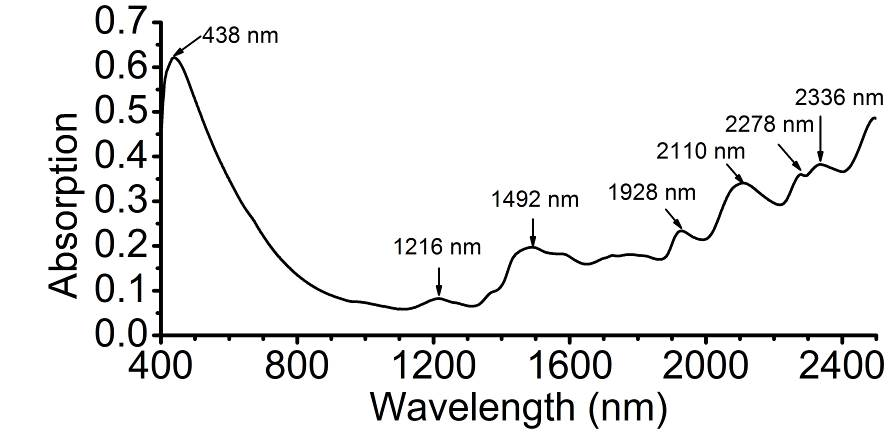Fig. 1. Averaged visible-near infrared spectrum of original data of 100 rice samples. The main absorption band peaks are pointed out by arrows

In terms of the distribution and variability of the spectral population and identification of anomalous samples, linearly uncorrelated components were extracted to characterize the 100 averaged spectra. Consequently, the total of 16 components and the first three components explained 99.45% and 90% of the variance in the spectral data, respectively. Based on the calculated Global “H” values (GH), none of the samples was identified as an outlier (i.e. GH ≥ 3), while most of the GH values ranged between 0.25 and 2.0 (Fig. 3a). The distribution of PCA data was acceptably symmetrical in the 3D plot (Fig. 3b), which indicated that the spectral were appropriate for VIS/NIR spectra analysis.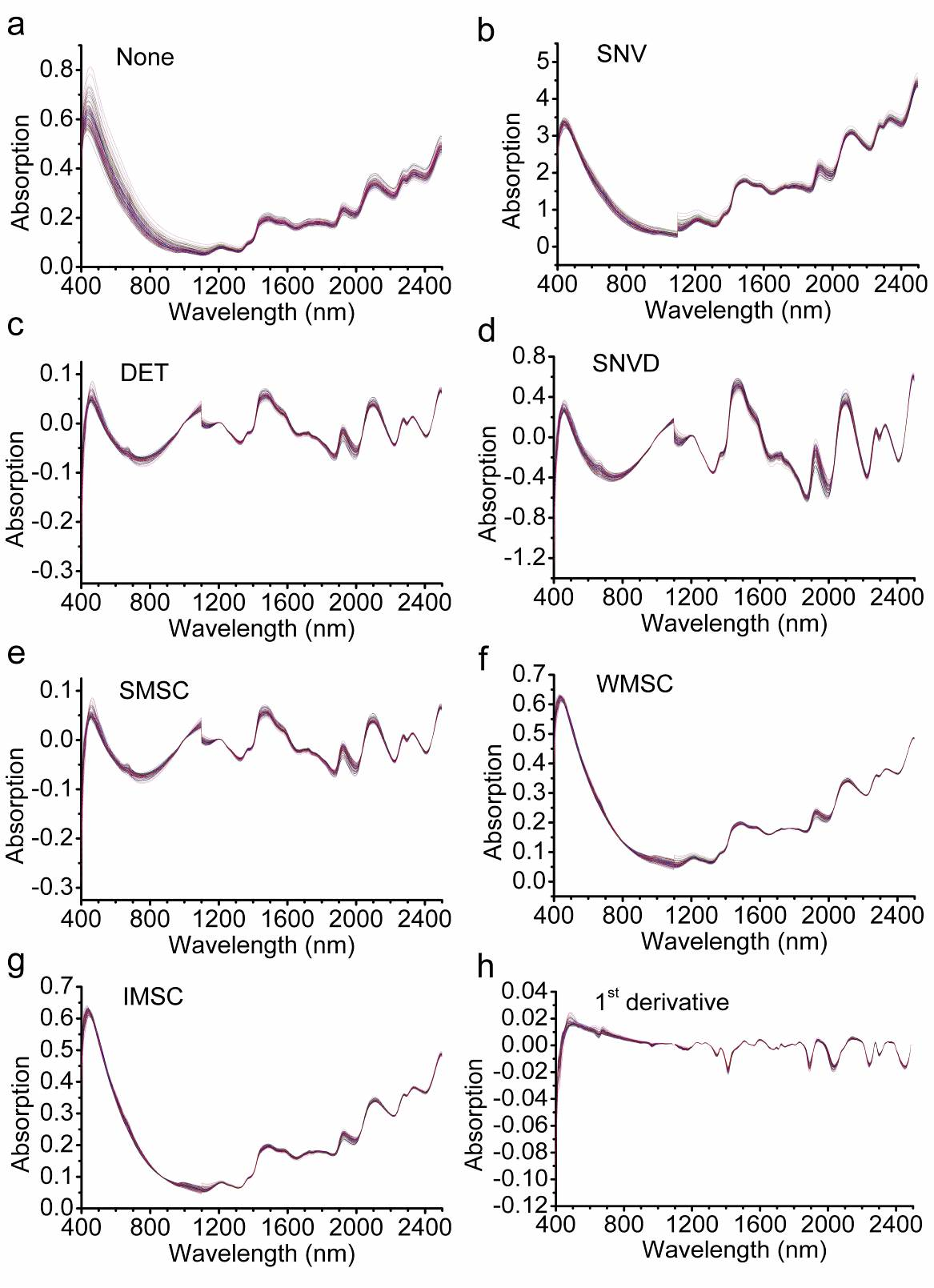Fig. 2. The original spectra and pretreated spectra of rice samples: (a) original spectra; (b) standard normal variant (SNV) pretreated spectra; (c) detrend (DET) pretreated spectra; (d) combination of SNV and detrend (SNVD) pretreated spectra; (e) standard multiple scatter correction (SMSC) pretreated spectra; (f) weighted multiple scatter correction (WMSC) pretreated spectra; (g) inverse multiple scatter correction (IMSC) pretreated spectra; and (h) 1st derivative pretreated spectra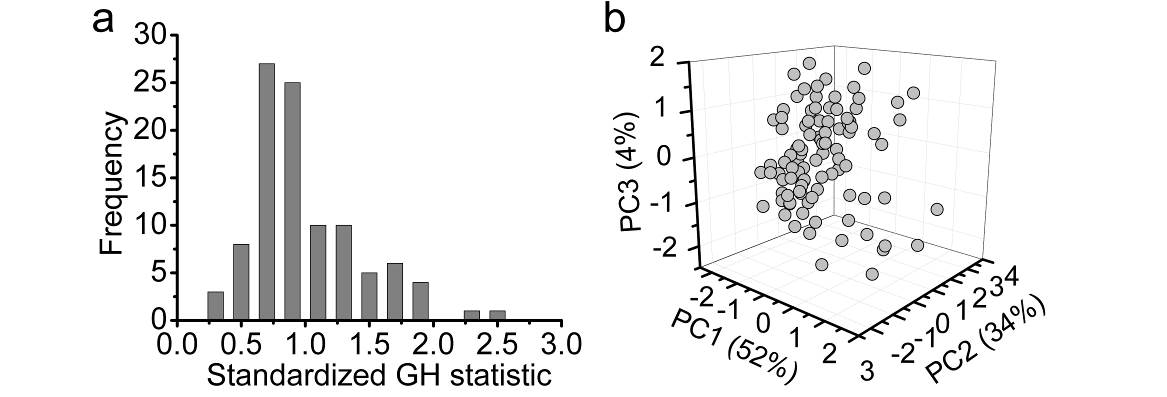Fig. 3. Histogram of global H (GH) value of 100 averaged spectra (a) and the three-dimensional plot of the PCA scores of spectra in principal components space (b)

Reference Data and Determination of Calibration/ External Validation Sets

Minimum and maximum contents of the three major lignin monomers (H, S, and G) measured/observed in the samples are presented in Table 1. The results showed that G represented the most abundant lignin unit in rice straw, followed by S. The total lignin monomers represented 2.4% of the cell wall material, which was consistent to previous studies that the thioacidolysis method released approximately 20% by weight of the lignin and the lignin levels in rice were approximately 11% to 19% of the dry matter basis (Jourdes et al. 2007; Li et al. 2015). Both the lignin monomers and the total monomer displayed high levels of diversity with the coefficient of variation (CV) were about 20% and relatively normal distribution (Table 1, Fig. 4). The substantial variation of these traits showed that the rice straw samples were suitable for NIRS assay.

Table 1. Statistics of the Lignin Monomer Contents of Rice Samples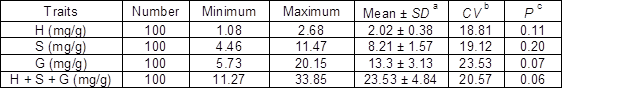Note: a Average ± standard deviation; b Coefficient of variation (SD*100/mean); P value of Kolmogorov-Smirnov; > 0.05 means the data are normally distributed

Based on the order of reference value, one of every four samples was selected and merged into the validation sets, and the remaining samples were included in the calibration sets. Thus, the external validation sets included 25 samples, and the calibration sets contained 75 samples. The trait values of the samples in the calibration and external validation sets displayed comparable range and similar standard deviation (Table 2). Moreover, samples in the external validation set were distributed evenly in the corresponding calibration samples as displayed in the three-dimensional plot (Fig. 5). Thus, the calibration and external validation sets were suitable for building and verifying equations.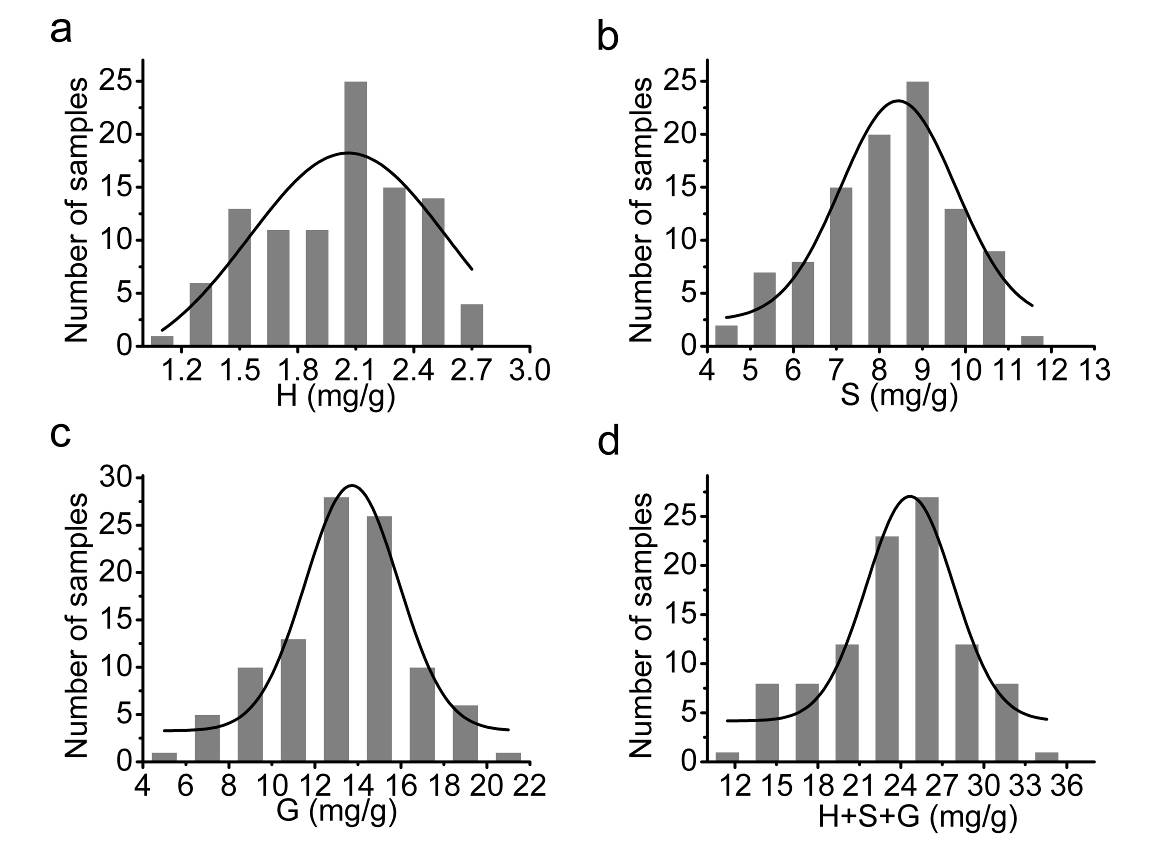Fig. 4. Frequency distributions of lignin monomer content in rice samples; frequency distribution was fitted by a Gaussian curve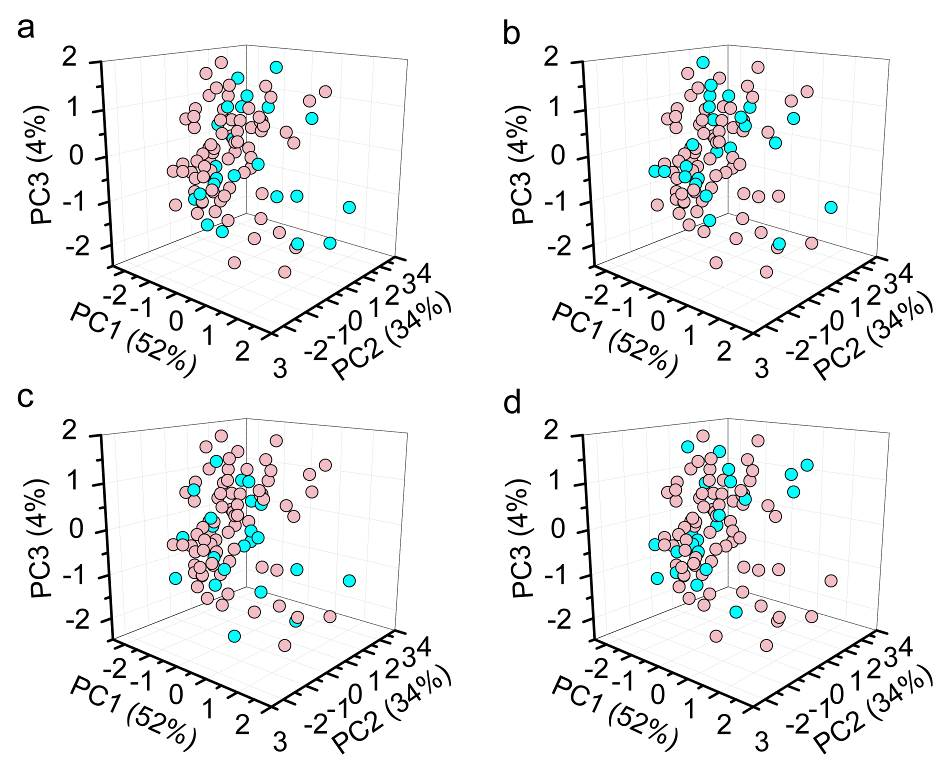Fig. 5. Distribution of the calibration set and external validation set for H (a), S (b), G (c), and H + S + G (d) in principal components space; the purple and blue circle represents the calibration and external validation set, respectively

Table 2. Calibration and External Validation Sets for Lignin Monomer Contents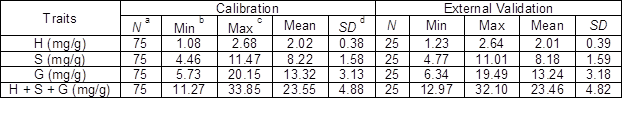a N– sample number; b Min- minimum value; c Max- maximum value; SD– standard deviation

Table 3. Coefficient of Determination for Cross-validation (R2cv) of Models Developed Using Different Spectrum Ranges and Mathematical Treatments for Lignin Monomers in Rice Materials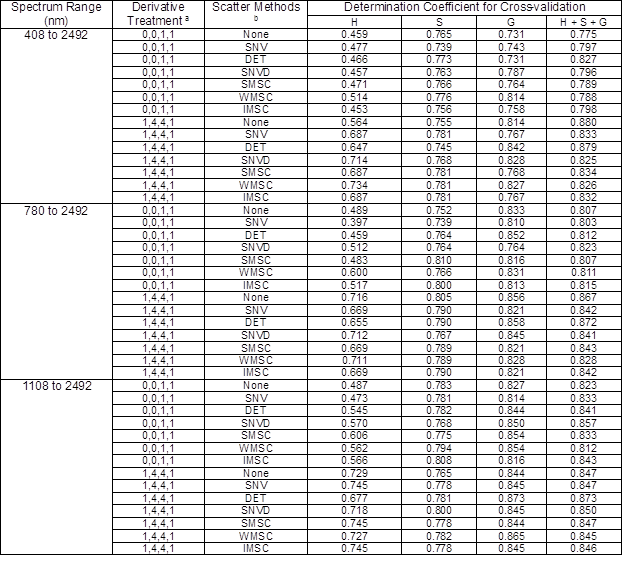The four digits orderly represent the number of the derivative, the gap over which the derivative was calculated, the number of the first smoothing, and the number of the second smoothing

None, no scatter correction standard; SNV, standard normal variant; DET, detrend only; SNVD, combination of SNV and detrend; SMSC, standard multiple scatter correction; WMSC, weighted multiple scatter correction; IMSC, inverse multiple scatter correction

Calibration and Validation of Lignin Monomeric Models

For the correlation between the spectral data and the laboratory values, seven scatter correction methods, two derivative treatments, and three spectrum regions combining the MPLS regression technique were applied to produce a total of 42 calibration equations for each trait (Table 3). The best equations according to the R2cv for the four traits were selected (Table 4). Generally speaking, no single math treatment or spectrum regions gave the best prediction for all traits. For H, G, and H + S + G, the best equation came from the 1, 4, 4, 1 derivative treatment using 6 factors, while the best equation for S came from the 0, 0, 1, 1 derivative treatment using 8 factors. Unlike single lignin monomers, the total lignin monomer was predicted best using the whole wavelength range including the visible spectrum, which indicated that the color of the rice powder might contain the information of the total lignin monomer content. The best equations for all four traits had high R2 values that ranged from 0.85 for H and 0.93 for H + S + G in calibration. In cross-validation, the equations also displayed acceptable R2cv values from 0.75 to 0.88 and ratio performance deviation (RPD) values from 2 to 3.01 (Arana et al. 2005). The external validation for the equations was conducted using the “Monitor results” module of the WinISI III software. The R2ev value of equations for these four traits ranged from 0.82 to 0.88 (Table 4). In addition, the predicted and reference values of these four traits showed good correlations, as indicated in the plots (Fig. 6) and the corresponding table of regression descriptors (Table 5).

Table 4. Calibration and Validation for the Best Equations Generated for Prediction of Lignin Monomer Contents in Rice Samples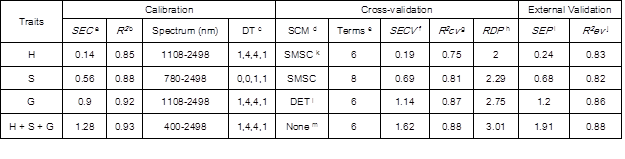a SEC, standard error of calibration; b R2, determination coefficient of calibration

c DT, derivative treatment; the four digits orderly represent the number of the derivative, the gap over which the derivative was calculated, the number of the first smoothing, and the number of the second smoothing

SCM, scatter correction methods

e Terms, number of principal component used for calibration

f SECV, standard error of cross-validation

g R2cv, determination coefficient of cross-validation

h RPD, ratio performance deviation (SD/SECV)

i SEP, standard error of prediction in external validation

j R2ev, determination coefficient of external validation

k SMSC, standard multiple scatter correction; DET, detrend only

m None, no scatter correction

Table 5. Descriptors of Regression Line between Measured and Predicted Values of Calibration Set and External Validation Set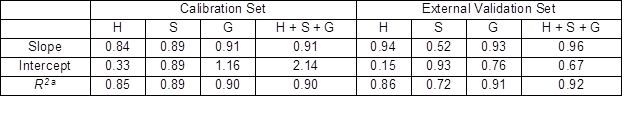a Coefficient of determination, represents the degree of fit of the regression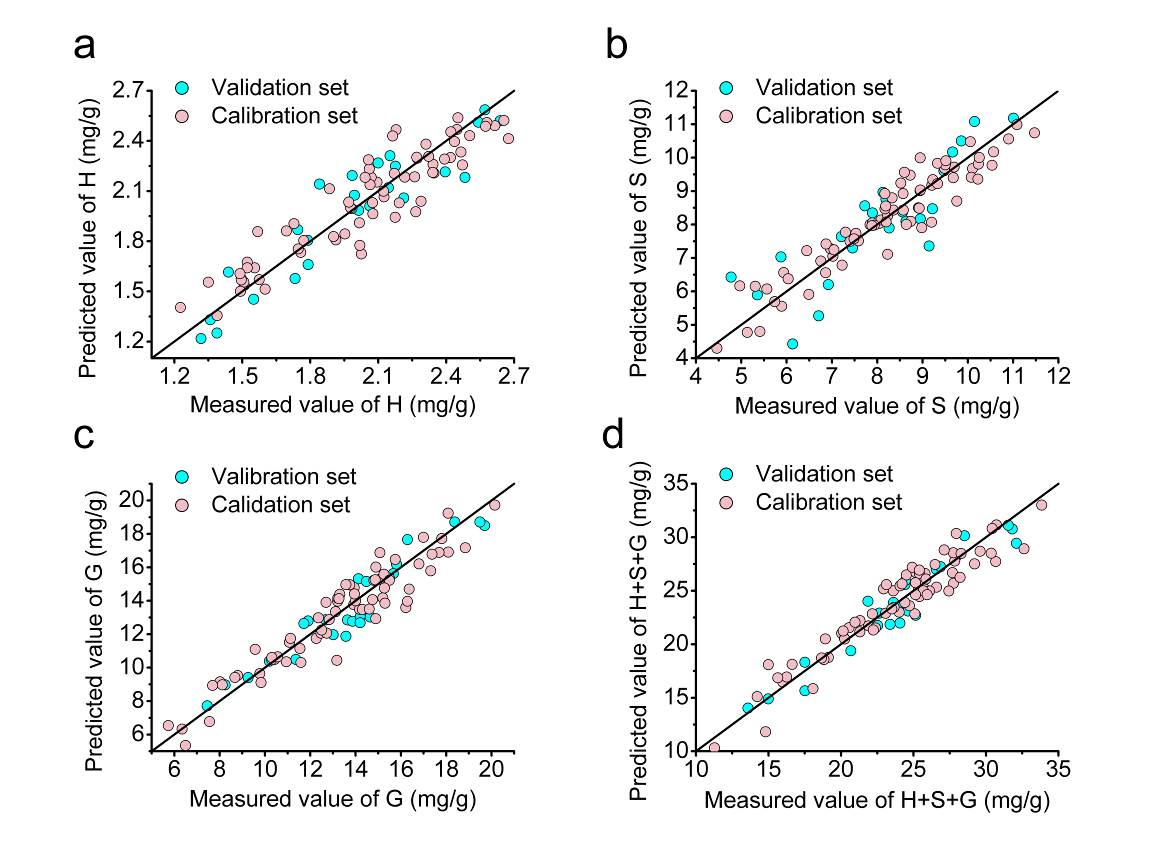Fig. 6. Correlation between the predicted and measured values of external validation set and calibration set for H (a), S (b), G (c), and H + S + G (d); the solid line indicates the best linear relationship (1:1)

The regression coefficients that could be used to gain insight on the molecular features underlying the best calibration models are displayed in Fig. 7. The strong correlation coefficients were found at wavelengths centered at 1636 nm and 1676 nm for H, 1668 nm and 2268 nm for S, 1660 nm, 2172 nm, 2364 nm, and 2444 nm for G, and 1652 nm, 1676 nm, 2164 nm, and 2380 nm for H + S + G.

In identified wavelength, the regression correlations at 1660 nm and 1668 nm were consistent with the chemical structure of lignin, as the bands at these wavelengths correspond to the first overtone of aromatic C-H stretching in lignin (Barton et al.1992; Michell and Schimleck 1996).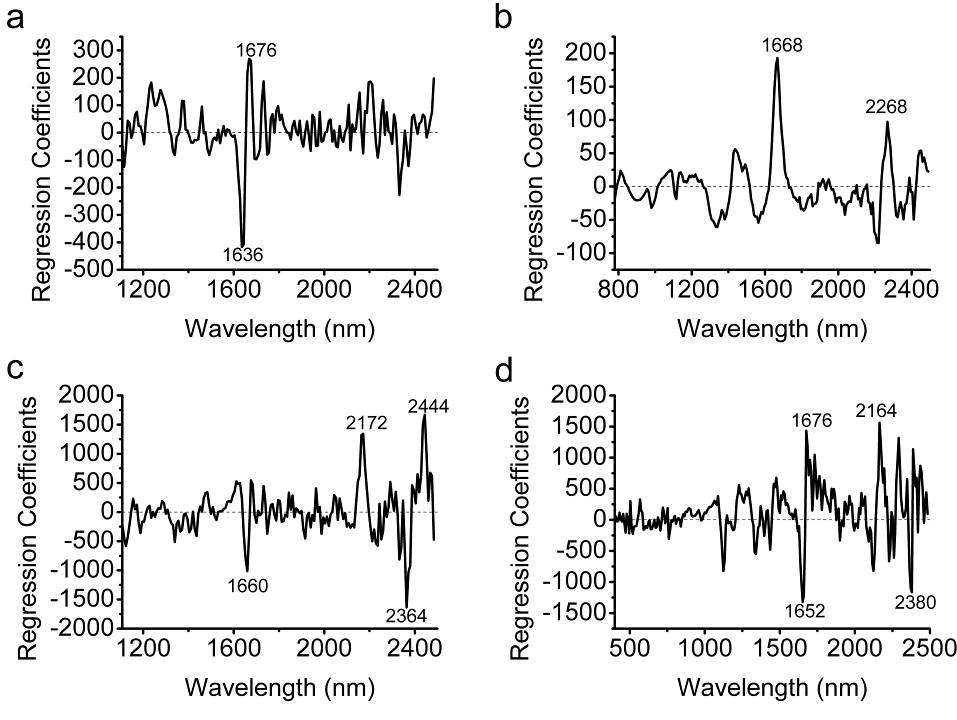Fig. 7. Corresponding plots of regression coefficients for the prediction model for H (a), S (b), G (c), and H + S + G (d); the main peaks are delineated

To investigate whether the accuracy of these equations were satisfied for QTL analysis, QTL mapping was conducted using the measured data and predicted data of 87 rice samples whose genotype data had been investigated. The logarithm of odds (LOD) scores of genetic loci calculated by two sets of data for all traits were similar in full genetic map (Fig. 8), indicating the equations were sufficiently accurate to generate phenotype data for mapping genetic loci controlling lignin composition in rice straw.